Question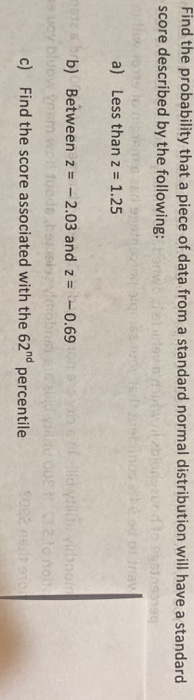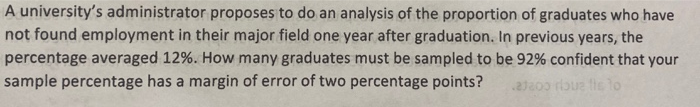1).a).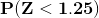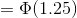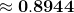[ using normal table]

b).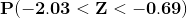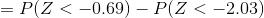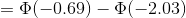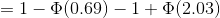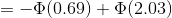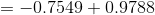[ using normal table]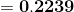c).let z be the 62nd percentile.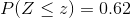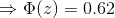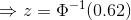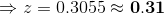the score for 62nd percentile = 0.31

2).the given data are:-

margin of error (E) = 2% = 0.02

proportion (p) = 0.12

z critical value for 92% confidence level, both tailed test be:-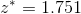[ in excel type =NORMSINV(0.96)]

the needed sample size be:-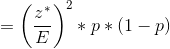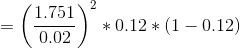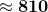*** if you have any doubt regarding the problem please write it in the comment box.if you are satisfied please give me a LIKE if possible...

#### Earn Coins

Coins can be redeemed for fabulous gifts.

Similar Homework Help Questions
• ### 17. Find the probability that a piece of data from a standard normal distribution will have...

17. Find the probability that a piece of data from a standard normal distribution will have a standard score described by the following: a) Less than z = 1.25 b) Between z = – 2.03 and z = – 0.69 c) Find the score associated with the 62nd percentile

• ### A university’s administrator proposes to do an analysis of the proportion of graduates who have not...

A university’s administrator proposes to do an analysis of the proportion of graduates who have not found employment in their major field one year after graduation. In previous years, the percentage averaged 12%. How many graduates must be sampled to be 92% confident that your sample percentage has a margin of error of two percentage points? if it can draw graph i need a graph too and i need clear write thank you for help

• ### Standard Normal distribution. With regards to a standard normal distribution complete the following: (a) Find P(Z...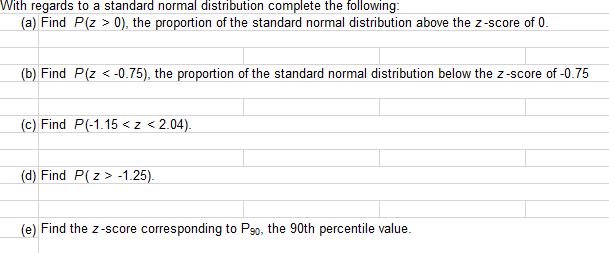Standard Normal distribution. With regards to a standard normal distribution complete the following: (a) Find P(Z > 0), the proportion of the standard normal distribution above the z-score of 0. (b) Find P(Z <-0.75), the proportion of the standard normal distribution below the Z-score of -0.75 (c) Find P(-1.15<z <2.04). (d) Find P(Z > -1.25). (e) Find the Z-score corresponding to Pso, the 90th percentile value.

• ### Find the proportion of observations from a standard normal distribution curve that satisfies z-score: -0.2<z< 0.6...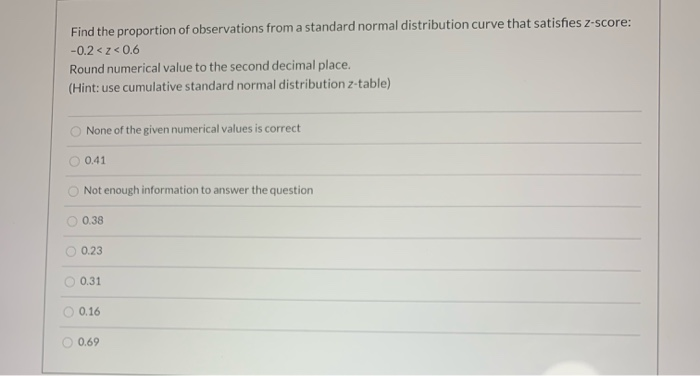Find the proportion of observations from a standard normal distribution curve that satisfies z-score: -0.2<z< 0.6 Round numerical value to the second decimal place, (Hint: use cumulative standard normal distribution z-table) None of the given numerical values is correct 0.41 Not enough information to answer the question 0.38 0.23 0.31 0.16 0.69

• ### 1. Use Table A to find the proportion of observations from a standard Normal distribution that...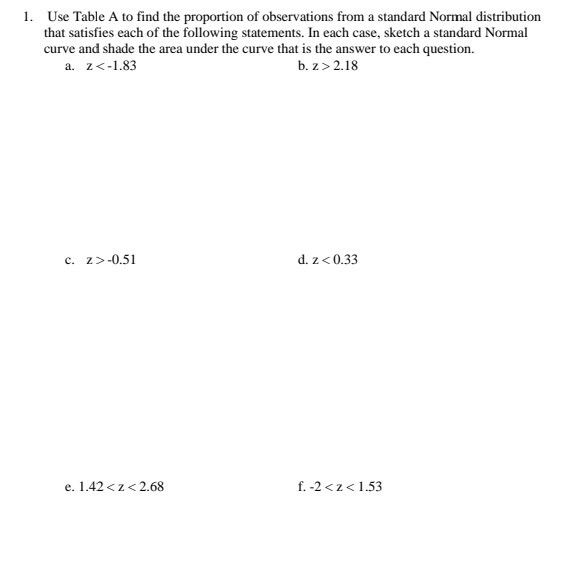1. Use Table A to find the proportion of observations from a standard Normal distribution that satisfies each of the following statements. In each case, sketch a standard Normal curve and shade the area under the curve that is the answer to each question. a. z<-1.83 b.z > 2.18 c. Z>-0.51 d. z <0.33 e. 1.42<z <2.68 f.-2 <2<1.53 X is a normally distributed variable with mean u = 30 and standard deviation o = 4. Find the following: a....